# 九、多视图几何

• 针孔相机模型校准
• 鱼眼镜头模型校准
• 立体相机校准 - 外在性估计
• 失真点和不失真点
• 消除图像中的镜头失真效果
• 通过三角测量从两个观测值还原 3D 点
• 通过 PnP 算法找到相对的相机对象姿态
• 通过立体校正对齐两个视图
• 对极几何 - 计算基本和本质矩阵
• 将基本矩阵分解为旋转和平移
• 估计立体图像的视差图
• 特例 2 - 视图几何 - 估计单应变换
• 平面场景 - 将单应性分解为旋转和平移
• 旋转相机 CAS - 从单应性估计相机旋转

# 怎么做

1. 导入必要的模块：
import cv2
import numpy as np

1. 从相机捕获帧，检测每个帧上的棋盘图案，并累积帧和角，直到我们有足够多的样本：
cap = cv2.VideoCapture(0)

pattern_size = (10, 7)

samples = []

while True:
if not ret:
break

res, corners = cv2.findChessboardCorners(frame, pattern_size)

img_show = np.copy(frame)
cv2.drawChessboardCorners(img_show, pattern_size, corners, res)
cv2.putText(img_show, 'Samples captured: %d' % len(samples), (0,
40),
cv2.FONT_HERSHEY_SIMPLEX, 1.0, (0, 255, 0), 2)
cv2.imshow('chessboard', img_show)

wait_time = 0 if res else 30
k = cv2.waitKey(wait_time)

if k == ord('s') and res:
samples.append((cv2.cvtColor(frame, cv2.COLOR_BGR2GRAY),
corners))
elif k == 27:
break

cap.release()
cv2.destroyAllWindows()

1. 使用cv2.cornerSubPix细化所有检测到的角点：
criteria = (cv2.TERM_CRITERIA_EPS + cv2.TERM_CRITERIA_MAX_ITER, 30, 1e-3)

for i in range(len(samples)):
img, corners = samples[i]
corners = cv2.cornerSubPix(img, corners, (10, 10), (-1,-1), criteria)

1. 通过将所有精确的角点传递到cv2.calibrateCamera来找到相机的固有参数：
pattern_points = np.zeros((np.prod(pattern_size), 3), np.float32)
pattern_points[:, :2] = np.indices(pattern_size).T.reshape(-1, 2)

images, corners = zip(*samples)

pattern_points = [pattern_points]*len(corners)

rms, camera_matrix, dist_coefs, rvecs, tvecs = \
cv2.calibrateCamera(pattern_points, corners, images.shape,
None, None)

np.save('camera_mat.npy', camera_matrix)
np.save('dist_coefs.npy', dist_coefs)


# 这个怎么运作

• 收集大量数据，例如图像和检测到的棋盘图案
• 提炼棋盘边角坐标
• 优化相机参数以使其与观察到的变形和投影相匹配

• 灰度图像
• 检测到的角点的大致坐标
• 细化区域的大小以找到更准确的角点位置
• 细化区域中心的区域大小，可以忽略
• 停止提炼过程的标准

• 所有样本的对象点坐标
• 所有样本的角点坐标
• （宽度，高度）格式的图像形状
• 两个数组保存平移和旋转向量（可以设置为 None）
• 标志和停止条件（均具有默认值）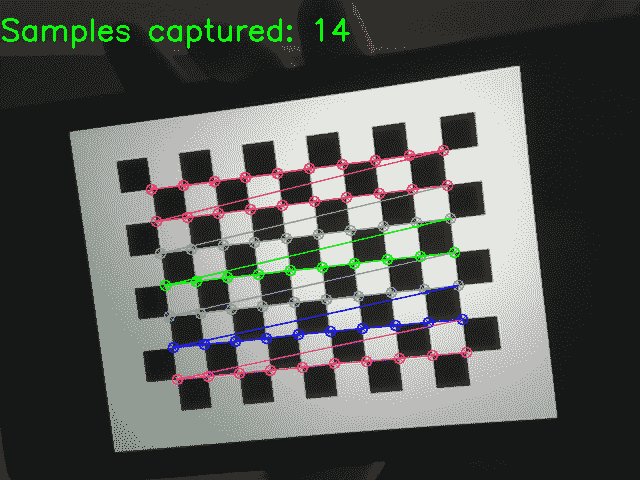# 怎么做

1. 导入必要的模块：
import cv2
import numpy as np

1. 从相机捕获帧，检测每个帧上的棋盘图案，并累积帧和角，直到我们有足够多的样本：
cap = cv2.VideoCapture(0)

pattern_size = (10, 7)

samples = []

while True:
if not ret:
break

res, corners = cv2.findChessboardCorners(frame, pattern_size)

img_show = np.copy(frame)
cv2.drawChessboardCorners(img_show, pattern_size, corners, res)
cv2.putText(img_show, 'Samples captured: %d' % len(samples), (0, 40),
cv2.FONT_HERSHEY_SIMPLEX, 1.0, (0, 255, 0), 2)
cv2.imshow('chessboard', img_show)

wait_time = 0 if res else 30
k = cv2.waitKey(wait_time)

if k == ord('s') and res:
samples.append((cv2.cvtColor(frame, cv2.COLOR_BGR2GRAY), corners))
elif k == 27:
break

cap.release()
cv2.destroyAllWindows()

1. 使用cv2.cornerSubPix细化所有检测到的角点：
criteria = (cv2.TERM_CRITERIA_EPS + cv2.TERM_CRITERIA_MAX_ITER, 30, 1e-3)

for i in range(len(samples)):
img, corners = samples[i]
corners = cv2.cornerSubPix(img, corners, (10, 10), (-1,-1), criteria)

1. 导入必要的模块，打开输入图像，然后复制它：
pattern_points = np.zeros((1, np.prod(pattern_size), 3), np.float32)
pattern_points[0, :, :2] = np.indices(pattern_size).T.reshape(-1, 2)

images, corners = zip(*samples)

pattern_points = [pattern_points]*len(corners)

print(len(pattern_points), pattern_points.shape, pattern_points.dtype)
print(len(corners), corners.shape, corners.dtype)

rms, camera_matrix, dis t_coefs, rvecs, tvecs = \
cv2.fisheye.calibrate(pattern_points, corners, images.shape, None, None)

np.save('camera_mat.npy', camera_matrix)
np.save('dist_coefs.npy', dist_coefs)


# 这个怎么运作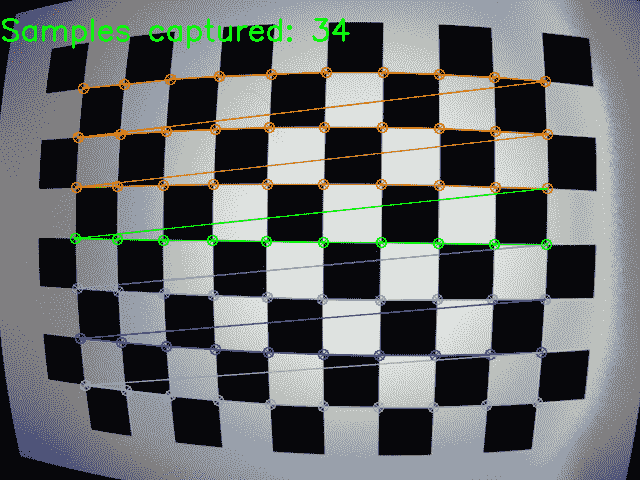# 怎么做

1. 导入必要的模块：
import cv2
import glob
import numpy as np

1. 设置图案大小并准备带有图像的列表：
PATTERN_SIZE = (9, 6)
left_imgs = list(sorted(glob.glob('../data/stereo/case1/left*.png')))
right_imgs = list(sorted(glob.glob('../data/stereo/case1/right*.png')))
assert len(left_imgs) == len(right_imgs)

1. 找到棋盘点：
criteria = (cv2.TERM_CRITERIA_EPS + cv2.TERM_CRITERIA_MAX_ITER, 30, 1e-3)
left_pts, right_pts = [], []
img_size = None

for left_img_path, right_img_path in zip(left_imgs, right_imgs):
if img_size is None:
img_size = (left_img.shape, left_img.shape)

res_left, corners_left = cv2.findChessboardCorners(left_img, PATTERN_SIZE)
res_right, corners_right = cv2.findChessboardCorners(right_img, PATTERN_SIZE)

corners_left = cv2.cornerSubPix(left_img, corners_left, (10, 10), (-1,-1),
criteria)
corners_right = cv2.cornerSubPix(right_img, corners_right, (10, 10), (-1,-1),
criteria)

left_pts.append(corners_left)
right_pts.append(corners_right)

1. 准备校准图案点：
pattern_points = np.zeros((np.prod(PATTERN_SIZE), 3), np.float32)
pattern_points[:, :2] = np.indices(PATTERN_SIZE).T.reshape(-1, 2)
pattern_points = [pattern_points] * len(left_imgs)

1. 估计立体对参数：
err, Kl, Dl, Kr, Dr, R, T, E, F = cv2.stereoCalibrate(
pattern_points, left_pts, right_pts, None, None, None, None, img_size, flags=0)

1. 报告校准结果：
print('Left camera:')
print(Kl)
print('Left camera distortion:')
print(Dl)
print('Right camera:')
print(Kr)
print('Right camera distortion:')
print(Dr)
print('Rotation matrix:')
print(R)
print('Translation:')
print(T)


# 这个怎么运作

Left camera:
[[ 534.36681752    0\.          341.45684657]
[   0\.          534.29616718  235.72519106]
[   0\.            0\.            1\.        ]]
Left camera distortion:
[[ -2.79470900e-01   4.71876981e-02   1.39511507e-03  -1.64158448e-04
7.01729203e-02]]
Right camera:
[[ 537.88729748    0\.          327.29925115]
[   0\.          537.43063947  250.10021993]
[   0\.            0\.            1\.        ]]
Right camera distortion:
[[-0.28990693  0.12537789 -0.00040656  0.00053461 -0.03844589]]
Rotation matrix:
[[ 0.99998995  0.00355598  0.00273003]
[-0.00354058  0.99997791 -0.00562461]
[-0.00274997  0.00561489  0.99998046]]
Translation:
[[-3.33161159]
[ 0.03706722]
[-0.00420814]]


# 怎么做

1. 导入必要的模块：
import cv2
import numpy as np

1. 加载相机的相机矩阵和失真系数：
camera_matrix = np.load('../data/pinhole_calib/camera_mat.npy')

1. 打开相机拍摄的国际象棋棋盘的照片，然后找到并优化角点：
img = cv2.imread('../data/pinhole_calib/img_00.png')
pattern_size = (10, 7)
res, corners = cv2.findChessboardCorners(img, pattern_size)
criteria = (cv2.TERM_CRITERIA_EPS + cv2.TERM_CRITERIA_MAX_ITER, 30, 1e-3)
corners = cv2.cornerSubPix(cv2.cvtColor(img, cv2.COLOR_BGR2GRAY),
corners, (10, 10), (-1,-1), criteria)

1. 取消扭曲角的坐标并将其转换为 3D 点：
h_corners = cv2.undistortPoints(corners, camera_matrix, dist_coefs)
h_corners = np.c_[h_corners.squeeze(), np.ones(len(h_corners))]

1. 将角点的 3D 坐标投影到图像上而不应用失真：
img_pts, _ = cv2.projectPoints(h_corners, (0, 0, 0), (0, 0, 0), camera_matrix, None)

for c in corners:
cv2.circle(img, tuple(c), 10, (0, 255, 0), 2)

for c in img_pts.squeeze().astype(np.float32):
cv2.circle(img, tuple(c), 5, (0, 0, 255), 2)

cv2.imshow('undistorted corners', img)
cv2.waitKey()
cv2.destroyAllWindows()

1. 将角点的 3D 坐标投影到图像上并应用镜头变形：
img_pts, _ = cv2.projectPoints(h_corners, (0, 0, 0), (0, 0, 0), camera_matrix, dist_coefs)

for c in img_pts.squeeze().astype(np.float32):
cv2.circle(img, tuple(c), 2, (255, 255, 0), 2)

cv2.imshow('reprojected corners', img)
cv2.waitKey()
cv2.destroyAllWindows()


# 这个怎么运作

cv2.undistortPoints查找图像中各点的同类坐标。 此函数消除了镜头变形并投影了点，使其处于无量纲坐标。 该函数接受以下参数：图像中的 2D 点数组，3x3相机矩阵，一组失真系数，用于存储结果的对象以及在立体视觉中使用的校正和投影矩阵，并且现在无所谓。 最后三个参数是可选的。 cv2.undistortPoints返回未失真和未投影点的集合。

cv2.undistortPoints返回的点是理想的-它们的坐标是无量纲的，并且不会因镜头而失真。 如果需要将它们投影回去，则需要将它们转换为 3D 点。 为此，我们只需要向每个点添加第三个Z坐标即可。 由于这些点的坐标是同质的，因此Z等于 1。

cv2.>projectPoints的参数包括：某些局部坐标系中的 3D 点数组，从局部坐标系到相机坐标系的转换的旋转和平移向量，3x3相机矩阵，失真系数数组，用于存储结果点的对象，用于存储 Jacobian 值的对象以及宽高比的值。 同样，最后三个参数是可选的，可以省略。 此函数返回 3D 点和 Jacobian 值的投影坐标和变形坐标。 如果要获取没有透镜变形的点的位置，则可以将None传递为变形系数数组的值。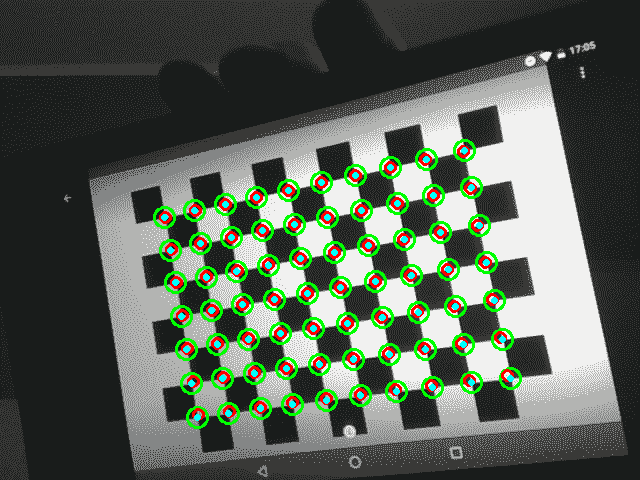# 怎么做

1. 导入必要的模块：
import cv2
import numpy as np

1. 加载同一相机拍摄的相机矩阵和畸变系数以及照片：
camera_matrix = np.load('../data/pinhole_calib/camera_mat.npy')

1. 使用cv2.undistort取消扭曲图像-图像中将出现空白区域：
ud_img = cv2.undistort(img, camera_matrix, dist_coefs)

cv2.imshow('undistorted image', ud_img)
cv2.waitKey(0)

cv2.destroyAllWindows()

1. 通过计算最佳相机矩阵并将其应用以获得没有黑色区域的未经失真的图像来消除空白区域：
opt_cam_mat, valid_roi = cv2.getOptimalNewCameraMatrix(camera_matrix, dist_coefs, img.shape[:2][::-1], 0)

ud_img = cv2.undistort(img, camera_matrix, dist_coefs, None, opt_cam_mat)

cv2.imshow('undistorted image', ud_img)
cv2.waitKey(0)

cv2.destroyAllWindows()


# 这个怎么运作

cv2.undistort消除图像中的镜头失真。 它采用以下参数：要失真的图像，相机矩阵，失真系数数组，存储未失真图像的对象以及最佳相机矩阵。 最后两个参数是可选的。 该函数返回未失真的图像。 如果错过了cv2.undistort的最后一个参数，则生成的图像将包含空白区域（黑色）。 最佳摄影机矩阵参数可让您获得没有这些伪影的图像，但是我们需要一种计算此最佳摄影机矩阵的方法，OpenCV 会为其提供服务。

cv2.getOptimalNewCameraMatrix创建最佳相机矩阵，以消除未失真图像上的黑色区域。 它需要相机矩阵，失真系数，（宽度，高度）格式的原始图像大小，alpha 因子，所得图像大小（同样的（宽度，高度）格式），以及设置摄像机的主要相机点在输出图像的中心的布尔标志。 最后两个参数是可选的。 alpha 因子是[0. 1]范围内的两倍，它表示删除空白区域的程度：0 表示完全删除，因此损失了一部分图像像素，而 1 表示保留了所有图像像素。 以及空白区域。 如果未设置输出图像尺寸，则将其设置为与输入图像的尺寸相同。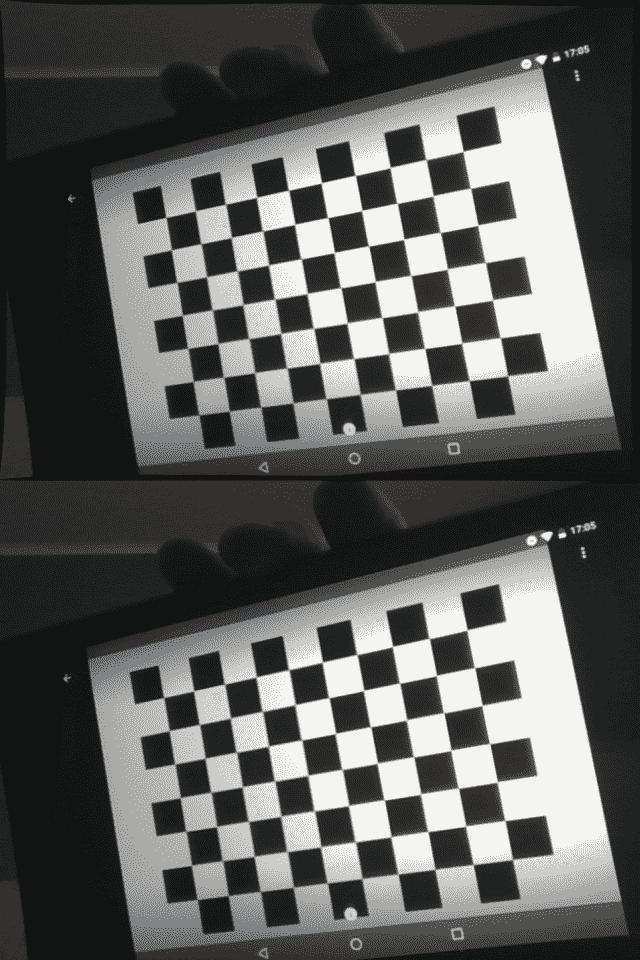# 怎么做

1. 导入必要的模块。
import cv2
import numpy as np

1. 生成测试相机的投影矩阵：
P1 = np.eye(3, 4, dtype=np.float32)
P2 = np.eye(3, 4, dtype=np.float32)
P2[0, 3] = -1

1. 生成测试点：
N = 5
points3d = np.empty((4, N), np.float32)
points3d[:3, :] = np.random.randn(3, N)
points3d[3, :] = 1

1. 将 3D 点投影到两个视图中并添加噪点：
points1 = P1 @ points3d
points1 = points1[:2, :] / points1[2, :]
points1[:2, :] += np.random.randn(2, N) * 1e-2

points2 = P2 @ points3d
points2 = points2[:2, :] / points2[2, :]
points2[:2, :] += np.random.randn(2, N) * 1e-2

1. 从嘈杂的观察中重建点：
points3d_reconstr = cv2.triangulatePoints(P1, P2, points1, points2)
points3d_reconstr /= points3d_reconstr[3, :]

1. 打印结果：
print('Original points')
print(points3d[:3].T)
print('Reconstructed points')
print(points3d_reconstr[:3].T)


# 这个怎么运作

Original points
[[ 0.48245686 -2.05779004  1.3458606 ]
[-0.18333936 -1.00662899 -0.46047512]
[-0.51193094 -0.54561883  0.20674749]
[ 1.05258393 -1.55241323  0.60368073]
[ 1.80103588 -0.83367926 -0.59293056]]
Reconstructed points
[[ 0.47777811 -2.05873108  1.3407315 ]
[-0.17389734 -0.99433696 -0.45361272]
[-0.51100874 -0.54552656  0.20692034]
[ 1.05780101 -1.54776227  0.60341281]
[ 1.81407869 -0.83914387 -0.59897166]]


# 怎么做

1. 导入必要的模块：
import cv2
import numpy as np

1. 加载相机矩阵，失真系数和相机拍摄的对象的照片：
camera_matrix = np.load('../data/pinhole_calib/camera_mat.npy')

1. 检测图像中的对象点，在本例中为棋盘角：
pattern_size = (10, 7)
res, corners = cv2.findChessboardCorners(img, pattern_size)
criteria = (cv2.TERM_CRITERIA_EPS + cv2.TERM_CRITERIA_MAX_ITER, 30, 1e-3)
corners = cv2.cornerSubPix(cv2.cvtColor(img, cv2.COLOR_BGR2GRAY),
corners, (10, 10), (-1,-1), criteria)

1. 创建 3D 对象点的配置：
pattern_points = np.zeros((np.prod(pattern_size), 3), np.float32)
pattern_points[:, :2] = np.indices(pattern_size).T.reshape(-1, 2)

1. 使用cv2.solvePnP查找对象的位置和方向：
ret, rvec, tvec = cv2.solvePnP(pattern_points, corners, camera_matrix, dist_coefs,
None, None, False, cv2.SOLVEPNP_ITERATIVE)

1. 通过应用找到的旋转和平移，将对象的点投影回图像。 绘制投影点：
img_points, _ = cv2.projectPoints(pattern_points, rvec, tvec, camera_matrix, dist_coefs)

for c in img_points.squeeze():
cv2.circle(img, tuple(c), 10, (0, 255, 0), 2)

cv2.imshow('points', img)
cv2.waitKey()

cv2.destroyAllWindows()


# 这个怎么运作

cv2.solvePnP能够通过对象在本地坐标系中的 3D 点及其在图像上的 2D 投影找到对象的平移和旋转。 它接受一组 3D 点，一组 2D 点，一个3x3相机矩阵，畸变系数，初始旋转和平移向量（可选），是否使用初始位置和方向的标记以及问题求解器的类型 。 前两个参数应包含相同数量的点。 求解器的类型可以是许多类型之一：cv2.SOLVEPNP_ITERATIVEcv2.SOLVEPNP_EPNPcv2.SOLVEPNP_DLS等。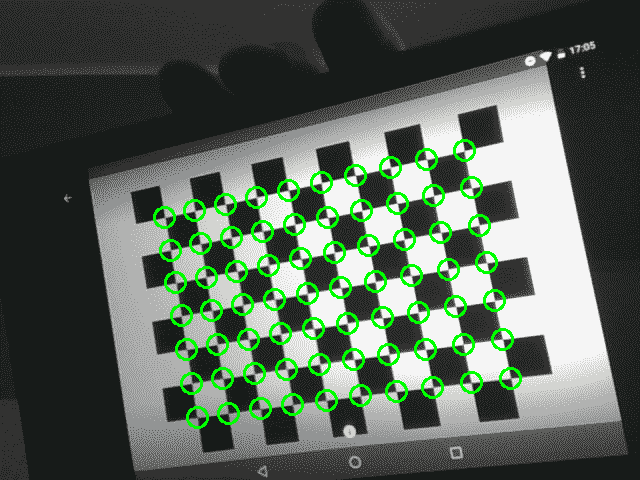# 怎么做

1. 导入必要的模块：
import cv2
import numpy as np
import matplotlib.pyplot as plt

1. 加载立体相机校准参数：
data = np.load('../data/stereo/case1/stereo.npy').item()
Kl, Dl, Kr, Dr, R, T, img_size = data['Kl'], data['Dl'], data['Kr'], data['Dr'], \
data['R'], data['T'], data['img_size']

1. 加载左右测试图像：
left_img = cv2.imread('../data/stereo/case1/left14.png')

1. 估计立体整流参数：
R1, R2, P1, P2, Q, validRoi1, validRoi2 = cv2.stereoRectify(Kl, Dl, Kr, Dr,
img_size, R, T)

1. 准备立体整流变换图：
xmap1, ymap1 = cv2.initUndistortRectifyMap(Kl, Dl, R1, Kl, img_size, cv2.CV_32FC1)
xmap2, ymap2 = cv2.initUndistortRectifyMap(Kr, Dr, R2, Kr, img_size, cv2.CV_32FC1)

1. 纠正图像：
left_img_rectified = cv2.remap(left_img, xmap1, ymap1, cv2.INTER_LINEAR)
right_img_rectified = cv2.remap(right_img, xmap2, ymap2, cv2.INTER_LINEAR)

1. 可视化结果：
plt.figure(0, figsize=(12,10))
plt.subplot(221)
plt.title('left original')
plt.imshow(left_img, cmap='gray')
plt.subplot(222)
plt.title('right original')
plt.imshow(right_img, cmap='gray')
plt.subplot(223)
plt.title('left rectified')
plt.imshow(left_img_rectified, cmap='gray')
plt.subplot(224)
plt.title('right rectified')
plt.imshow(right_img_rectified, cmap='gray')
plt.tight_layout()
plt.show()


# 这个怎么运作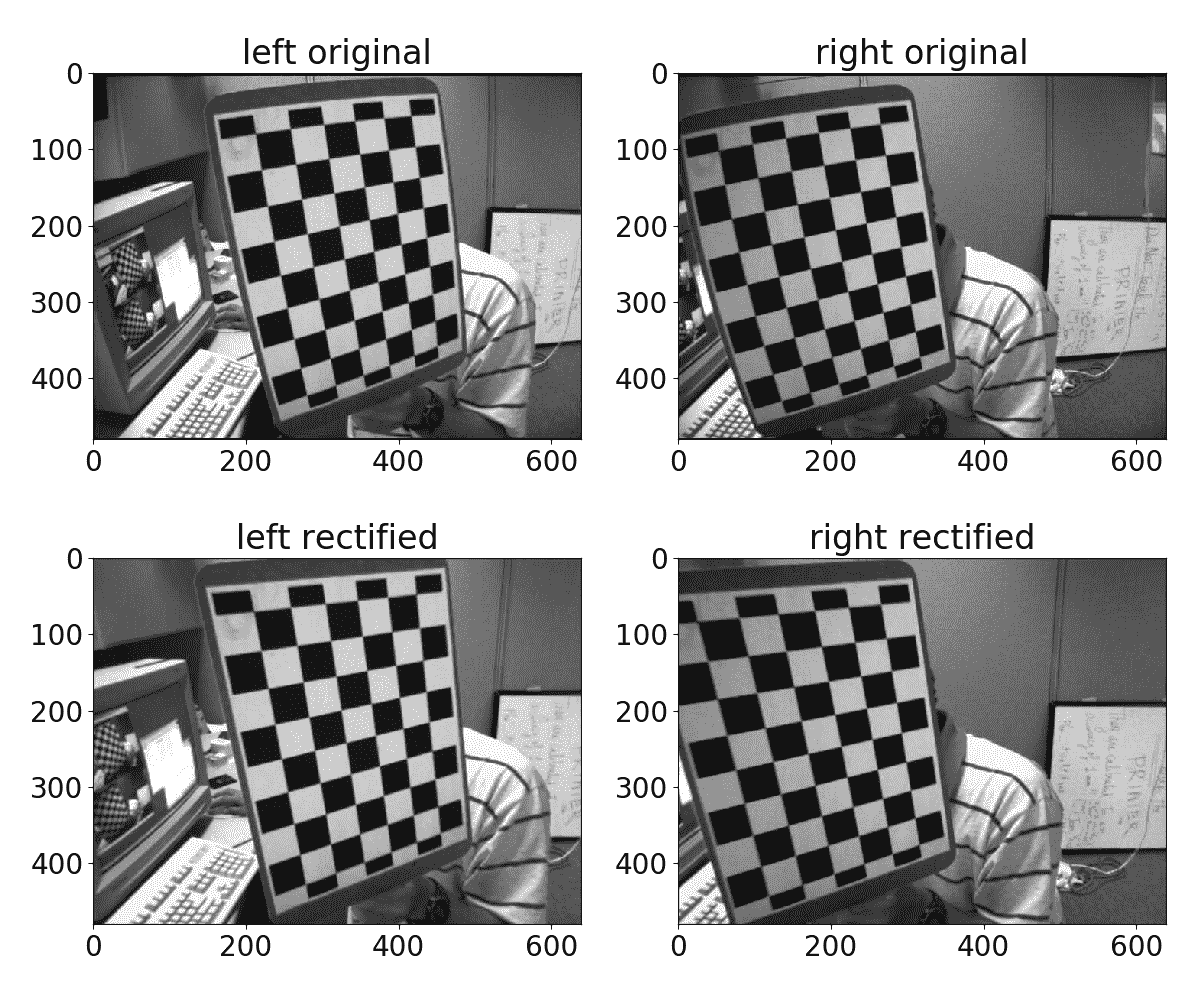# 怎么做

1. 导入必要的模块：
import cv2
import numpy as np

1. 加载左/右图像点对应关系和各个相机校准参数：
data = np.load('../data/stereo/case1/stereo.npy').item()
Kl, Kr, Dl, Dr, left_pts, right_pts, E_from_stereo, F_from_stereo = \
data['Kl'], data['Kr'], data['Dl'], data['Dr'], \
data['left_pts'], data['right_pts'], data['E'], data['F']

1. 将左右点列表堆叠到数组中：
left_pts = np.vstack(left_pts)
right_pts = np.vstack(right_pts)

1. 消除镜头变形：
left_pts = cv2.undistortPoints(left_pts, Kl, Dl, P=Kl)
right_pts = cv2.undistortPoints(right_pts, Kr, Dr, P=Kr)

1. 估计基本矩阵：
F, mask = cv2.findFundamentalMat(left_pts, right_pts, cv2.FM_LMEDS)

1. 估计本质矩阵：
E = Kr.T @ F @ Kl

1. 打印结果：
print('Fundamental matrix:')
print(F)
print('Essential matrix:')
print(E)


# 这个怎么运作

Fundamental matrix:
[[  1.60938825e-08  -2.23906409e-06  -2.53850603e-04]
[  2.97226703e-06  -2.38236386e-07  -7.70276666e-02]
[ -2.55190056e-04   7.69760820e-02   1.00000000e+00]]
Essential matrix:
[[  4.62585055e-03  -6.43487140e-01  -4.17486092e-01]
[  8.53590806e-01  -6.84088948e-02  -4.08817705e+01]
[  2.63679084e-01   4.07046349e+01  -2.20825664e-01]]


# 怎么做

1. 导入必要的模块：
import cv2
import numpy as np

1. 加载预先计算的基本矩阵：
data = np.load('../data/stereo/case1/stereo.npy').item()
E = data['E']

1. 将基本矩阵分解为两个可能的旋转和平移：
R1, R2, T = cv2.decomposeEssentialMat(E)

1. 打印结果：
print('Rotation 1:')
print(R1)
print('Rotation 2:')
print(R2)
print('Translation:')
print(T)


# 这个怎么运作

Rotation 1:
[[ 0.99981105 -0.01867927  0.00538031]
[-0.01870903 -0.99980965  0.00553437]
[ 0.00527591 -0.00563399 -0.99997021]]
Rotation 2:
[[ 0.99998995  0.00355598  0.00273003]
[-0.00354058  0.99997791 -0.00562461]
[-0.00274997  0.00561489  0.99998046]]
Translation:
[[ 0.99993732]
[-0.01112522]
[ 0.00126302]]


# 怎么做

1. 导入必要的模块：
import cv2
import numpy as np

1. 加载左右校正图像：
left_img = cv2.imread('../data/stereo/left.png')

1. 使用立体块匹配算法计​​算视差图：
stereo_bm = cv2.StereoBM_create(32)
dispmap_bm = stereo_bm.compute(cv2.cvtColor(left_img, cv2.COLOR_BGR2GRAY),
cv2.cvtColor(right_img, cv2.COLOR_BGR2GRAY))

1. 使用立体半全局块匹配算法计​​算视差图：
stereo_sgbm = cv2.StereoSGBM_create(0, 32)
dispmap_sgbm = stereo_sgbm.compute(left_img, right_img)

1. 可视化结果：
plt.figure(figsize=(12,10))
plt.subplot(221)
plt.title('left')
plt.imshow(left_img[:,:,[2,1,0]])
plt.subplot(222)
plt.title('right')
plt.imshow(right_img[:,:,[2,1,0]])
plt.subplot(223)
plt.title('BM')
plt.imshow(dispmap_bm, cmap='gray')
plt.subplot(224)
plt.title('SGBM')
plt.imshow(dispmap_sgbm, cmap='gray')
plt.show()


# 这个怎么运作

OpenCV 中还有一个名为cudastereo的模块，该模块是通过 CUDA 支持构建的，该模块提供了更优化的立体算法。 您还可以在 OpenCV Contrib 存储库中检出stereo模块，该模块还包含一些其他算法。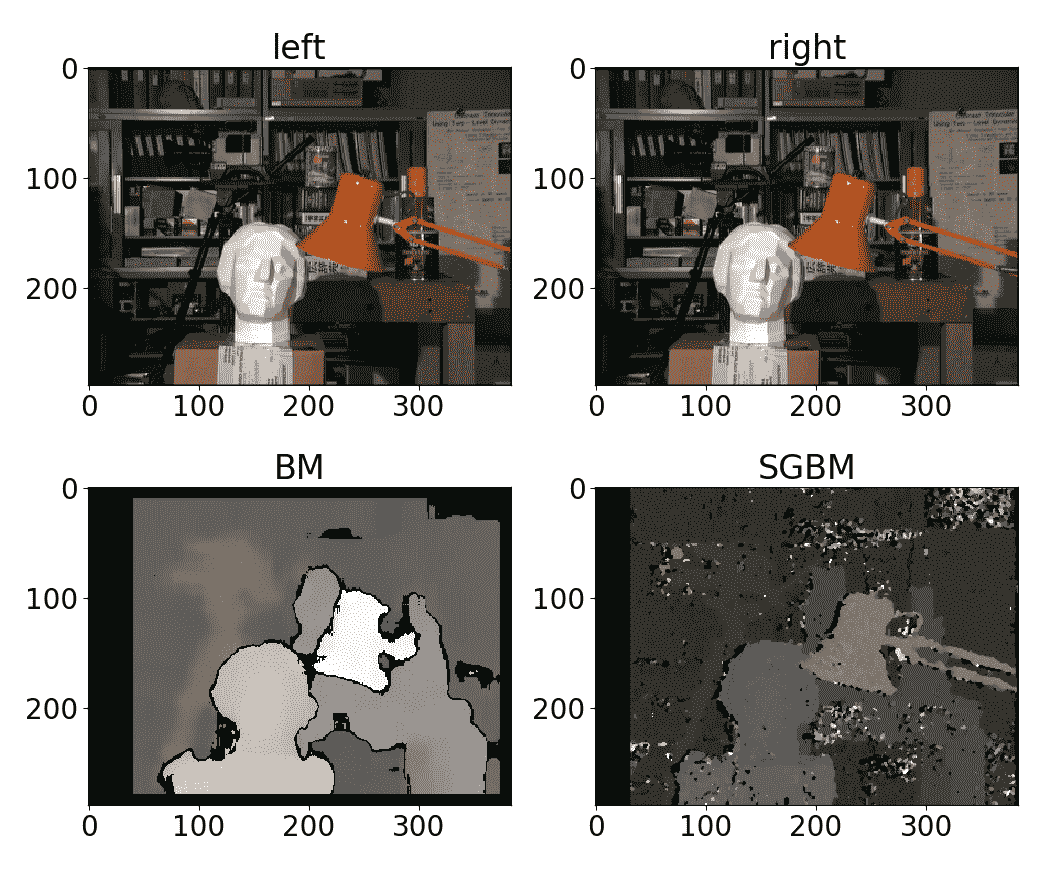# 怎么做

1. 导入必要的模块：
import cv2
import numpy as np

1. 加载相机矩阵，失真系数和相机拍摄的两帧：
camera_matrix = np.load('../data/pinhole_calib/camera_mat.npy')

1. 取消扭曲帧：
img_0 = cv2.undistort(img_0, camera_matrix, dist_coefs)
img_1 = cv2.undistort(img_1, camera_matrix, dist_coefs)

1. 在两个图像上找到棋盘角：
pattern_size = (10, 7)
res_0, corners_0 = cv2.findChessboardCorners(img_0, pattern_size)
res_1, corners_1 = cv2.findChessboardCorners(img_1, pattern_size)

criteria = (cv2.TERM_CRITERIA_EPS + cv2.TERM_CRITERIA_MAX_ITER, 30, 1e-3)
corners_0 = cv2.cornerSubPix(cv2.cvtColor(img_0, cv2.COLOR_BGR2GRAY),
corners_0, (10, 10), (-1,-1), criteria)
corners_1 = cv2.cornerSubPix(cv2.cvtColor(img_1, cv2.COLOR_BGR2GRAY),
corners_1, (10, 10), (-1,-1), criteria)

1. 在两个图像的点之间找到单应性：
H, mask = cv2.findHomography(corners_0, corners_1)

1. 应用找到的单应性矩阵将点从第一张图像投影到第二张图像：
center_0 = np.mean(corners_0.squeeze(), 0)
center_0 = np.r_[center_0, 1]
center_1 = H @ center_0
center_1 = (center_1 / center_1).astype(np.float32)

img_0 = cv2.circle(img_0, tuple(center_0[:2]), 10, (0, 255, 0), 3)
img_1 = cv2.circle(img_1, tuple(center_1[:2]), 10, (0, 0, 255), 3)

1. 使用找到的单应性矩阵变换第一张图像并显示结果：
img_0_warped = cv2.warpPerspective(img_0, H, img_0.shape[:2][::-1])

cv2.imshow('homography', np.hstack((img_0, img_1, img_0_warped)))
cv2.waitKey()
cv2.destroyAllWindows()


# 这个怎么运作

• 来自源（第一）平面的一组点
• 来自目标（第二个）平面的一组点
• 查找单应性的方法
• 过滤异常值的阈值
• 离群值的输出遮罩
• 最大迭代次数
• 置信度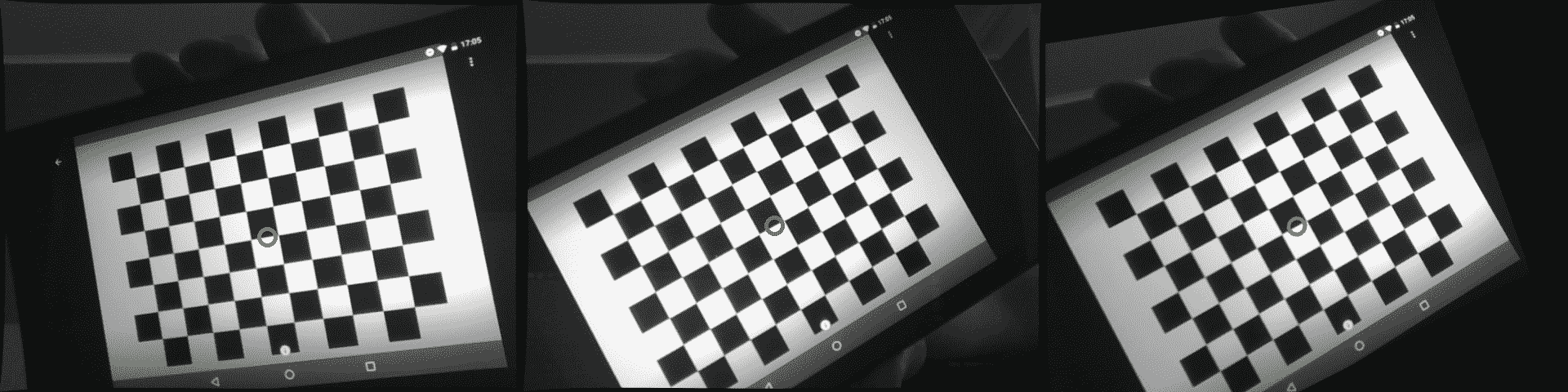# 怎么做

1. 导入必要的模块：
import cv2
import numpy as np

1. 加载相机矩阵，畸变系数和同一平面对象（棋盘图案）的两张照片。 然后，取消扭曲照片：
camera_matrix = np.load('../data/pinhole_calib/camera_mat.npy')
img_0 = cv2.undistort(img_0, camera_matrix, dist_coefs)
img_1 = cv2.undistort(img_1, camera_matrix, dist_coefs)

1. 在两个图像中找到图案的角点：
pattern_size = (10, 7)
res_0, corners_0 = cv2.findChessboardCorners(img_0, pattern_size)
res_1, corners_1 = cv2.findChessboardCorners(img_1, pattern_size)

criteria = (cv2.TERM_CRITERIA_EPS + cv2.TERM_CRITERIA_MAX_ITER, 30, 1e-3)
corners_0 = cv2.cornerSubPix(cv2.cvtColor(img_0, cv2.COLOR_BGR2GRAY),
corners_0, (10, 10), (-1,-1), criteria)
corners_1 = cv2.cornerSubPix(cv2.cvtColor(img_1, cv2.COLOR_BGR2GRAY),
corners_1, (10, 10), (-1,-1), criteria)

1. 找到从第一帧到第二帧的单应变换矩阵：
H, mask = cv2.findHomography(corners_0, corners_1)

1. 找到我们估计的单应矩阵的可能平移和旋转：
ret, rmats, tvecs, normals = cv2.decomposeHomographyMat(H, camera_matrix)


# 这个怎么运作

cv2.decomposeHomographyMat将单应性矩阵分解为旋转和平移。 由于解决方案不是唯一的，因此该函数最多返回四组可能的平移，旋转和法向向量集。 cv2.decomposeHomographyMat接受3x3单应矩阵和 3x3 摄影机矩阵作为参数。 返回值为：找到的解的数量，3x3旋转矩阵的列表，平移向量的列表和法线向量的列表。 每个返回的列表包含与找到的解决方案数量一样多的元素。

# 怎么做

1. 导入必要的模块：
import cv2
import numpy as np

1. 加载预先计算的单应性和相机参数：
data = np.load('../data/rotational_homography.npy').item()
H, K = data['H'], data['K']

1. 从单应性变换中考虑摄像机参数：
H_ = np.linalg.inv(K) @ H @ K

1. 计算近似旋转矩阵：
w, u, vt = cv2.SVDecomp(H_)
R = u @ vt
if cv2.determinant(R) < 0:
R *= 1

1. 将旋转矩阵转换为旋转向量：
rvec = cv2.Rodrigues(R)

1. 打印结果：
print('Rotation vector:')
print(rvec)


# 这个怎么运作

Rotation vector:
[[ 0.12439561]
[ 0.22688715]
[ 0.32641321]]